# `sklearn.linear_model`.SGDClassifier¶

class `sklearn.linear_model.``SGDClassifier`(loss='hinge', penalty='l2', alpha=0.0001, l1_ratio=0.15, fit_intercept=True, n_iter=5, shuffle=True, verbose=0, epsilon=0.1, n_jobs=1, random_state=None, learning_rate='optimal', eta0=0.0, power_t=0.5, class_weight=None, warm_start=False, average=False)[source]

Linear classifiers (SVM, logistic regression, a.o.) with SGD training.

This estimator implements regularized linear models with stochastic gradient descent (SGD) learning: the gradient of the loss is estimated each sample at a time and the model is updated along the way with a decreasing strength schedule (aka learning rate). SGD allows minibatch (online/out-of-core) learning, see the partial_fit method. For best results using the default learning rate schedule, the data should have zero mean and unit variance.

This implementation works with data represented as dense or sparse arrays of floating point values for the features. The model it fits can be controlled with the loss parameter; by default, it fits a linear support vector machine (SVM).

The regularizer is a penalty added to the loss function that shrinks model parameters towards the zero vector using either the squared euclidean norm L2 or the absolute norm L1 or a combination of both (Elastic Net). If the parameter update crosses the 0.0 value because of the regularizer, the update is truncated to 0.0 to allow for learning sparse models and achieve online feature selection.

Read more in the User Guide.

Parameters: loss : str, ‘hinge’, ‘log’, ‘modified_huber’, ‘squared_hinge’, ‘perceptron’, or a regression loss: ‘squared_loss’, ‘huber’, ‘epsilon_insensitive’, or ‘squared_epsilon_insensitive’ The loss function to be used. Defaults to ‘hinge’, which gives a linear SVM. The ‘log’ loss gives logistic regression, a probabilistic classifier. ‘modified_huber’ is another smooth loss that brings tolerance to outliers as well as probability estimates. ‘squared_hinge’ is like hinge but is quadratically penalized. ‘perceptron’ is the linear loss used by the perceptron algorithm. The other losses are designed for regression but can be useful in classification as well; see SGDRegressor for a description. penalty : str, ‘none’, ‘l2’, ‘l1’, or ‘elasticnet’ The penalty (aka regularization term) to be used. Defaults to ‘l2’ which is the standard regularizer for linear SVM models. ‘l1’ and ‘elasticnet’ might bring sparsity to the model (feature selection) not achievable with ‘l2’. alpha : float Constant that multiplies the regularization term. Defaults to 0.0001 Also used to compute learning_rate when set to ‘optimal’. l1_ratio : float The Elastic Net mixing parameter, with 0 <= l1_ratio <= 1. l1_ratio=0 corresponds to L2 penalty, l1_ratio=1 to L1. Defaults to 0.15. fit_intercept : bool Whether the intercept should be estimated or not. If False, the data is assumed to be already centered. Defaults to True. n_iter : int, optional The number of passes over the training data (aka epochs). The number of iterations is set to 1 if using partial_fit. Defaults to 5. shuffle : bool, optional Whether or not the training data should be shuffled after each epoch. Defaults to True. random_state : int seed, RandomState instance, or None (default) The seed of the pseudo random number generator to use when shuffling the data. verbose : integer, optional The verbosity level epsilon : float Epsilon in the epsilon-insensitive loss functions; only if loss is ‘huber’, ‘epsilon_insensitive’, or ‘squared_epsilon_insensitive’. For ‘huber’, determines the threshold at which it becomes less important to get the prediction exactly right. For epsilon-insensitive, any differences between the current prediction and the correct label are ignored if they are less than this threshold. n_jobs : integer, optional The number of CPUs to use to do the OVA (One Versus All, for multi-class problems) computation. -1 means ‘all CPUs’. Defaults to 1. learning_rate : string, optional The learning rate schedule: ‘constant’: eta = eta0 ‘optimal’: eta = 1.0 / (alpha * (t + t0)) [default] ‘invscaling’: eta = eta0 / pow(t, power_t) where t0 is chosen by a heuristic proposed by Leon Bottou. eta0 : double The initial learning rate for the ‘constant’ or ‘invscaling’ schedules. The default value is 0.0 as eta0 is not used by the default schedule ‘optimal’. power_t : double The exponent for inverse scaling learning rate [default 0.5]. class_weight : dict, {class_label: weight} or “balanced” or None, optional Preset for the class_weight fit parameter. Weights associated with classes. If not given, all classes are supposed to have weight one. The “balanced” mode uses the values of y to automatically adjust weights inversely proportional to class frequencies in the input data as `n_samples / (n_classes * np.bincount(y))` warm_start : bool, optional When set to True, reuse the solution of the previous call to fit as initialization, otherwise, just erase the previous solution. average : bool or int, optional When set to True, computes the averaged SGD weights and stores the result in the `coef_` attribute. If set to an int greater than 1, averaging will begin once the total number of samples seen reaches average. So `average=10` will begin averaging after seeing 10 samples. coef_ : array, shape (1, n_features) if n_classes == 2 else (n_classes, n_features) Weights assigned to the features. intercept_ : array, shape (1,) if n_classes == 2 else (n_classes,) Constants in decision function.

`LinearSVC`, `LogisticRegression`, `Perceptron`

Examples

```>>> import numpy as np
>>> from sklearn import linear_model
>>> X = np.array([[-1, -1], [-2, -1], [1, 1], [2, 1]])
>>> Y = np.array([1, 1, 2, 2])
>>> clf = linear_model.SGDClassifier()
>>> clf.fit(X, Y)
...
SGDClassifier(alpha=0.0001, average=False, class_weight=None, epsilon=0.1,
eta0=0.0, fit_intercept=True, l1_ratio=0.15,
learning_rate='optimal', loss='hinge', n_iter=5, n_jobs=1,
penalty='l2', power_t=0.5, random_state=None, shuffle=True,
verbose=0, warm_start=False)
>>> print(clf.predict([[-0.8, -1]]))

```

Methods

 `decision_function`(X) Predict confidence scores for samples. `densify`() Convert coefficient matrix to dense array format. `fit`(X, y[, coef_init, intercept_init, ...]) Fit linear model with Stochastic Gradient Descent. `fit_transform`(X[, y]) Fit to data, then transform it. `get_params`([deep]) Get parameters for this estimator. `partial_fit`(X, y[, classes, sample_weight]) Fit linear model with Stochastic Gradient Descent. `predict`(X) Predict class labels for samples in X. `score`(X, y[, sample_weight]) Returns the mean accuracy on the given test data and labels. `set_params`(\*args, \*\*kwargs) `sparsify`() Convert coefficient matrix to sparse format. `transform`(\*args, \*\*kwargs) DEPRECATED: Support to use estimators as feature selectors will be removed in version 0.19.
`__init__`(loss='hinge', penalty='l2', alpha=0.0001, l1_ratio=0.15, fit_intercept=True, n_iter=5, shuffle=True, verbose=0, epsilon=0.1, n_jobs=1, random_state=None, learning_rate='optimal', eta0=0.0, power_t=0.5, class_weight=None, warm_start=False, average=False)[source]
`decision_function`(X)[source]

Predict confidence scores for samples.

The confidence score for a sample is the signed distance of that sample to the hyperplane.

Parameters: X : {array-like, sparse matrix}, shape = (n_samples, n_features) Samples. array, shape=(n_samples,) if n_classes == 2 else (n_samples, n_classes) : Confidence scores per (sample, class) combination. In the binary case, confidence score for self.classes_ where >0 means this class would be predicted.
`densify`()[source]

Convert coefficient matrix to dense array format.

Converts the `coef_` member (back) to a numpy.ndarray. This is the default format of `coef_` and is required for fitting, so calling this method is only required on models that have previously been sparsified; otherwise, it is a no-op.

Returns: self: estimator :
`fit`(X, y, coef_init=None, intercept_init=None, sample_weight=None)[source]

Fit linear model with Stochastic Gradient Descent.

Parameters: X : {array-like, sparse matrix}, shape (n_samples, n_features) Training data y : numpy array, shape (n_samples,) Target values coef_init : array, shape (n_classes, n_features) The initial coefficients to warm-start the optimization. intercept_init : array, shape (n_classes,) The initial intercept to warm-start the optimization. sample_weight : array-like, shape (n_samples,), optional Weights applied to individual samples. If not provided, uniform weights are assumed. These weights will be multiplied with class_weight (passed through the constructor) if class_weight is specified self : returns an instance of self.
`fit_transform`(X, y=None, **fit_params)[source]

Fit to data, then transform it.

Fits transformer to X and y with optional parameters fit_params and returns a transformed version of X.

Parameters: X : numpy array of shape [n_samples, n_features] Training set. y : numpy array of shape [n_samples] Target values. X_new : numpy array of shape [n_samples, n_features_new] Transformed array.
`get_params`(deep=True)[source]

Get parameters for this estimator.

Parameters: deep : boolean, optional If True, will return the parameters for this estimator and contained subobjects that are estimators. params : mapping of string to any Parameter names mapped to their values.
`partial_fit`(X, y, classes=None, sample_weight=None)[source]

Fit linear model with Stochastic Gradient Descent.

Parameters: X : {array-like, sparse matrix}, shape (n_samples, n_features) Subset of the training data y : numpy array, shape (n_samples,) Subset of the target values classes : array, shape (n_classes,) Classes across all calls to partial_fit. Can be obtained by via np.unique(y_all), where y_all is the target vector of the entire dataset. This argument is required for the first call to partial_fit and can be omitted in the subsequent calls. Note that y doesn’t need to contain all labels in classes. sample_weight : array-like, shape (n_samples,), optional Weights applied to individual samples. If not provided, uniform weights are assumed. self : returns an instance of self.
`predict`(X)[source]

Predict class labels for samples in X.

Parameters: X : {array-like, sparse matrix}, shape = [n_samples, n_features] Samples. C : array, shape = [n_samples] Predicted class label per sample.
`predict_log_proba`

Log of probability estimates.

This method is only available for log loss and modified Huber loss.

When loss=”modified_huber”, probability estimates may be hard zeros and ones, so taking the logarithm is not possible.

See `predict_proba` for details.

Parameters: X : array-like, shape (n_samples, n_features) T : array-like, shape (n_samples, n_classes) Returns the log-probability of the sample for each class in the model, where classes are ordered as they are in self.classes_.
`predict_proba`

Probability estimates.

This method is only available for log loss and modified Huber loss.

Multiclass probability estimates are derived from binary (one-vs.-rest) estimates by simple normalization, as recommended by Zadrozny and Elkan.

Binary probability estimates for loss=”modified_huber” are given by (clip(decision_function(X), -1, 1) + 1) / 2. For other loss functions it is necessary to perform proper probability calibration by wrapping the classifier with `sklearn.calibration.CalibratedClassifierCV` instead.

Parameters: X : {array-like, sparse matrix}, shape (n_samples, n_features) array, shape (n_samples, n_classes) : Returns the probability of the sample for each class in the model, where classes are ordered as they are in self.classes_.

References

Zadrozny and Elkan, “Transforming classifier scores into multiclass probability estimates”, SIGKDD‘02, http://www.research.ibm.com/people/z/zadrozny/kdd2002-Transf.pdf

The justification for the formula in the loss=”modified_huber” case is in the appendix B in: http://jmlr.csail.mit.edu/papers/volume2/zhang02c/zhang02c.pdf

`score`(X, y, sample_weight=None)[source]

Returns the mean accuracy on the given test data and labels.

In multi-label classification, this is the subset accuracy which is a harsh metric since you require for each sample that each label set be correctly predicted.

Parameters: X : array-like, shape = (n_samples, n_features) Test samples. y : array-like, shape = (n_samples) or (n_samples, n_outputs) True labels for X. sample_weight : array-like, shape = [n_samples], optional Sample weights. score : float Mean accuracy of self.predict(X) wrt. y.
`sparsify`()[source]

Convert coefficient matrix to sparse format.

Converts the `coef_` member to a scipy.sparse matrix, which for L1-regularized models can be much more memory- and storage-efficient than the usual numpy.ndarray representation.

The `intercept_` member is not converted.

Returns: self: estimator :

Notes

For non-sparse models, i.e. when there are not many zeros in `coef_`, this may actually increase memory usage, so use this method with care. A rule of thumb is that the number of zero elements, which can be computed with `(coef_ == 0).sum()`, must be more than 50% for this to provide significant benefits.

After calling this method, further fitting with the partial_fit method (if any) will not work until you call densify.

`transform`(*args, **kwargs)[source]

DEPRECATED: Support to use estimators as feature selectors will be removed in version 0.19. Use SelectFromModel instead.

Reduce X to its most important features.

Uses `coef_` or `feature_importances_` to determine the most important features. For models with a `coef_` for each class, the absolute sum over the classes is used.
Parameters: X : array or scipy sparse matrix of shape [n_samples, n_features] The input samples. threshold : string, float or None, optional (default=None)The threshold value to use for feature selection. Features whose importance is greater or equal are kept while the others are discarded. If “median” (resp. “mean”), then the threshold value is the median (resp. the mean) of the feature importances. A scaling factor (e.g., “1.25*mean”) may also be used. If None and if available, the object attribute `threshold` is used. Otherwise, “mean” is used by default. X_r : array of shape [n_samples, n_selected_features] The input samples with only the selected features.

## Examples using `sklearn.linear_model.SGDClassifier`¶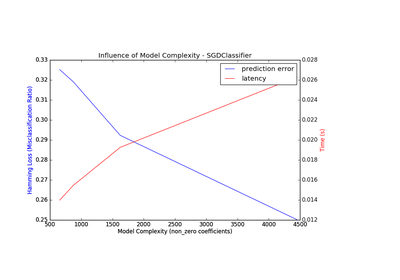Model Complexity Influence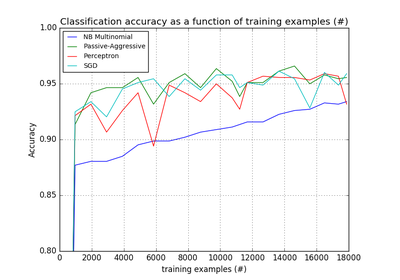Out-of-core classification of text documents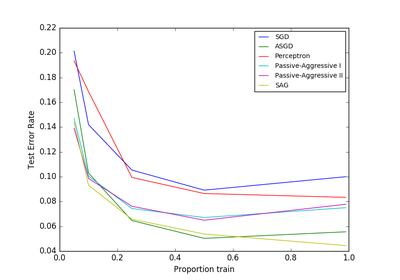Comparing various online solvers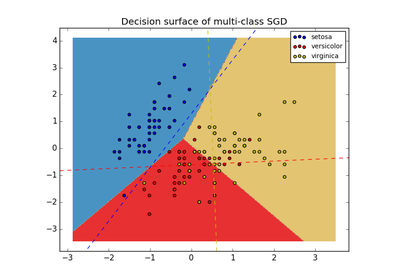Plot multi-class SGD on the iris dataset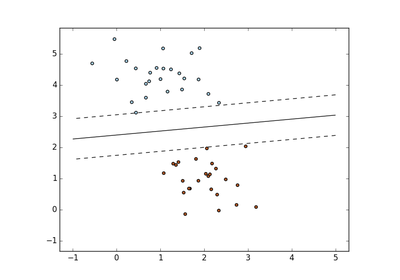SGD: Maximum margin separating hyperplane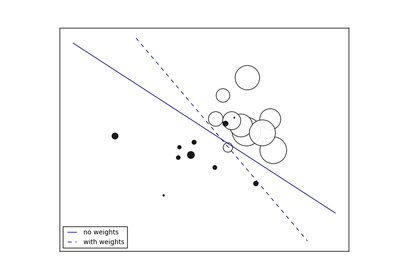SGD: Weighted samplesSample pipeline for text feature extraction and evaluationClassification of text documents using sparse featuresClassification of text documents: using a MLComp dataset